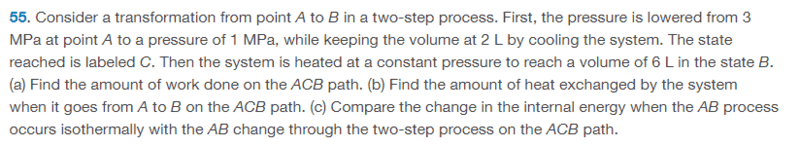# Heat exchanged in Expanding and cooling gas

• ChiralSuperfields

#### ChiralSuperfields

Homework Statement
Relevant Equations
For part(b),My solution is,
##\Delta E_{int} = Q - W = \frac{3}{2}(P_fV_f - P_iV_i)##
##Q = W + \frac{3}{2}(P_fV_f - P_iV_i)##
##Q = 4000 + \frac{3}{2}((1 \times 10^6)(6 \times 10^{-3}) - (3 \times 10^6)(2 \times 10^{-3})##
##Q = 4000 J##

However, according to the solution b. ##−4000 J##

Can someone please tell me what I did wrong?

Many thanks!

#### Attachments

I'm uncertain what would in general be meant by the amount of heat "exchanged" by a system. It could mean the amount gained, the amount lost, or the magnitude of the transfer (so positive). Looks like they intended the amount lost.

•ChiralSuperfields

•ChiralSuperfields
I'm uncertain what would in general be meant by the amount of heat "exchanged" by a system. It could mean the amount gained, the amount lost, or the magnitude of the transfer (so positive). Looks like they intended the amount lost.

If they intended for the amount lost, why dose the first law in my answer give the wrong sign? If you don't know, please don't worry about it, this textbook dose have a lot of mistakes!

Many thanks!

•